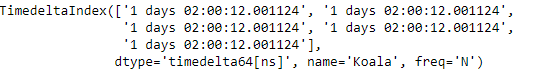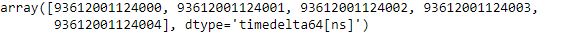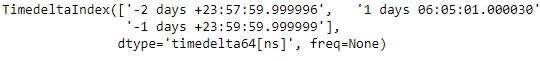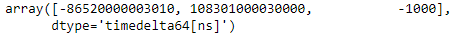Related Articles
Python | Pandas TimedeltaIndex.values
• Last Updated : 29 Dec, 2018

Python is a great language for doing data analysis, primarily because of the fantastic ecosystem of data-centric python packages. Pandas is one of those packages and makes importing and analyzing data much easier.

Pandas` TimedeltaIndex.values` attribute return the underlying data for the given TimedeltaIndex object as an ndarray.

Syntax : TimedeltaIndex.values

Return : ndarray

Example #1: Use `TimedeltaIndex.values` attribute to return the value of the given TimedeltaIndex object as an ndarray.

 `# importing pandas as pd``import` `pandas as pd`` ` `# Create the TimedeltaIndex object``tidx ``=` `pd.TimedeltaIndex(start ``=``'1 days 02:00:12.001124'``, periods ``=` `5``, ``                                            ``freq ``=``'N'``, name ``=``'Koala'``)`` ` `# Print the TimedeltaIndex``print``(tidx)`

Output :Now we will print the data of the given tidx object as an ndarray.

 `# return the data of tidx as an ndarray``tidx.values`

Output :As we can see in the output, the `TimedeltaIndex.values` attribute has returned the data of the tidx as an ndarray.

Example #2: Use `TimedeltaIndex.values` attribute to return the value of the given TimedeltaIndex object as an ndarray.

 `# importing pandas as pd``import` `pandas as pd`` ` `# Create the TimedeltaIndex object``tidx ``=` `pd.TimedeltaIndex(data ``=``[``'-1 days 2 min 3us 10ns'``, ``'1 days 06:05:01.000030'``,``                                                      ``'-1 days + 23:59:59.999999'``])`` ` `# Print the TimedeltaIndex``print``(tidx)`

Output :Now we will print the data of the given tidx object as an ndarray.

 `# return the data of tidx as an ndarray``tidx.values`

Output :As we can see in the output, the `TimedeltaIndex.values` attribute has returned the data of the tidx as an ndarray.

Attention geek! Strengthen your foundations with the Python Programming Foundation Course and learn the basics.

To begin with, your interview preparations Enhance your Data Structures concepts with the Python DS Course.

My Personal Notes arrow_drop_up